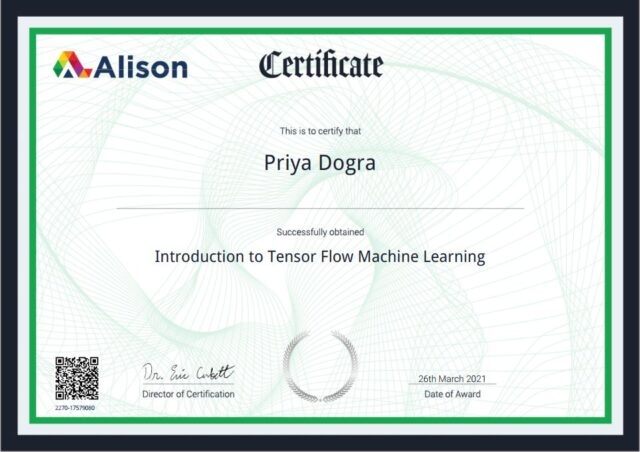Home Certification Introduction to Tensor Flow Machine Learning Course Assessment Answers – Alison

# Introduction to Tensor Flow Machine Learning Course Assessment Answers – Alison

0## Enroll Here: Introduction to Tensor Flow Machine Learning Certification

Question 1: TensorFlow was released under Apache 2.0 license in ___  2015. Drag correct answer to the box.

• May
• October
• January
• November

Question 2: A tensor is defined by the following attributes. Choose three.

• Number of axis
• Size
• Data type
• Shape

Question 3: 2D tensors are also known as ___. Fill in the blank.

Question 4: True or false. We can learn f(x) = 1/x using a neural network exactly.

• True
• False

Question 5: True or false. The government wants to build a model that predicts the water usage of a locality based on certain features. They start off with the model building process by collecting data from three major cities. To build a model that generalizes over all three cities, the best way to split the data is to take the data of two cities as the training data and the data of the third city as the test data.

• True
• False

Question 6: We are solving a multiclass classification with 6 classes. We have 4 features for each example in our data. There are two hidden layers with 6 units each. Each of the units use sigmoid activation. What are the total number of parameters in the neural network? Answer in numeric form.

Question 7: Which of the following statements are true? Choose two.

• Adding new features to the data always results in equal or better performance on the training set
• Increasing the number of layers and units in a neural network always results in equal or better performance in the training set
• Increasing the number of layers and units in a neural network always results in equal or better performance in the test set
• Adding new features to the data always results in equal or better performance on the test set

Question 8: Features and label are components of ___. Fill in the blank.

Question 9: True or false. TensorFlow is a vibrant active community of developers with 500 developers actively contributing to the code base.

• True
• False

Question 10: True or false. A neural network learns complex functions by breaking it down into simple functions.

• True
• False

Question 11: True or false. The tanh activation works better than sigmoid activation because of the mean of the output of tanh is closer to zero and hence it centres the data better for the next layer.

• True
• False

Question 12: Gradient descent consists of ___ steps.

• Seven
• Eleven
• Eight
• Six

Question 13: In ___,  you end up achieving a 0 error on the training data, but your model may not generalize well on the future data. Fill in the blank.

Question 14: We want to perform regression over training data (x_train, y_train) and test the model on (x_test, y_test). Suppose y_train and y_test come from the following distributions below. After training the model, which of the following situations is most likely to occur when we evaluate it on test data? Choose one.

• Low error when y_test = 0 and high error when y_test = 2
• Low error when y_test = 2 and high error when y_test = -2
• Low error when y_test = -3 and high error when y_test = 3
• Low error when y_test = 2 and high error when y_test = 1

Question 15: Select the correct value of f1_score(A) for this confusion matrix. Choose one.

• 0.5
• 0.0
• 0.75
• 1.0

Question 16: True or false. TensorFlow has a very rigid architecture.

• True
• False

Question 17: Given the following confusion matrix for a classification task with three classes (A, B and C), which of the following is correct? Choose two.

• Recall (B) = 1.0
• Precision (B) = 1.0
• Precision (C) = 1.0
• Recall (C) = 1.0

Question 18: Tensor Flow is an end-to-end open-source platform for ___ learning. Fill in the blank.

Question 19: TensorFlow is a vibrant active community of developers with more than ___ developers actively contributing to the code base. Answer in numeric form.

Question 20: True or false. One of the goals in machine learning is to build models that make good predictions on future data rather than on the past data.

• True
• False

Question 21: ___ metric is foolproof while evaluating the classification task? Drag correct answer to box.

• Accuracy
• F1 Score

Question 22: ___is the first and the most important aspect or prerequisite for a machine learning model. Fill in the blank.

Question 23: What is the range of average f1_score? Choose one.

• 0.6 – 0.79
• 0.8 – 1.0
• 0.4 – 0.59
• 0.2 – 0.39

Question 24: MNIST dataset contains grace skilled images of handwritten ___. Fill in the blank.

Question 25: 1D tensors are also known as ___. Fill in the blank.

Question 26: A situation where the model is not taken into account a training data at all is known as ___. Fill in the blank.

Question 27: Let f(x,y) = q(w1x + w2y + b), where x and y are binary inputs. Where can this function fit on? Choose three.

• XOR (x,y)
• AND (x,y)
• NOT(x)
• OR (x,y)

Question 28: There are ___ stages in the machine learning process. Fill in the blank.

• Three
• Seven
• Two
• Five

Question 29: True or false. We use a small percentage of data for training and hold out a large chunk of data for test.

• True
• False

Question 30: ___ activation function has actual returns, a number between plus -1 and +1. Fill in the blank.